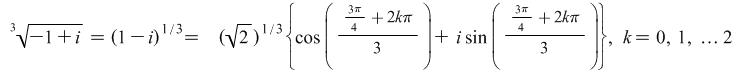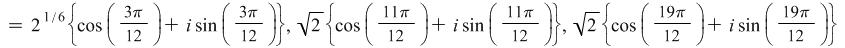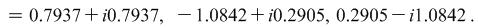Arithmetic Operations on Complex Numbers

Addition of two complex numbers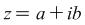and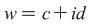is carried out thus: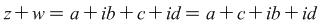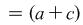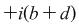.

The real part of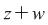is therefore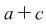and the imaginary part is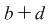.

In a similar way,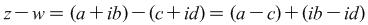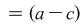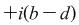.

The real part of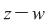is therefore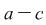and the imaginary part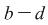.

## 1.1  Examples

If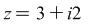and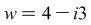what is

i)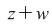ii)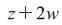Solutions:

i)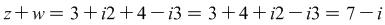.

ii)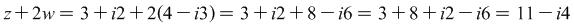.

# 2  Multiplication

Multiplication of two complex numbersandis carried out thus: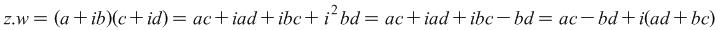.

The real part of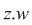is therefore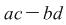and the imaginary part is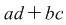.

## 2.1  Examples

Ifandwhat is

i)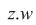ii)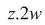Solutions:

i)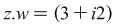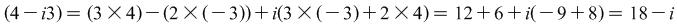.

ii)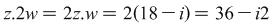.

# 3  Division

Division is achieved through use of the complex congugate,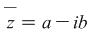, i.e.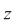with the sign of the imaginary part reversed. It will be noted that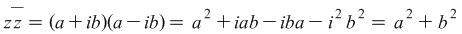i.e. a real, positive number.

Division of a complex number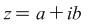by another complex numberis facilitated by multiplying both numerator and denominator by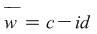giving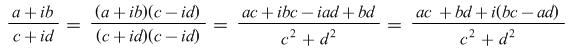.

## 3.1  Examples

Convert the fraction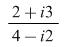to the form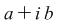.

Solution: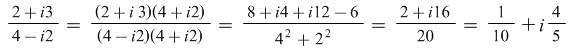.

# 4  Powers of complex numbers

Powers of pure imaginary numbers of the form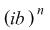are found by calculating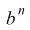and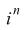separately and multiplying the two results together.

## 4.1  Example

Calculate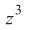where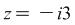.

Solution: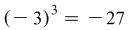.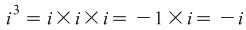.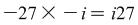.

Therefore,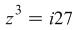.

Powers of complex numbers of the formcan be calculated by expanding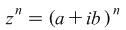. However, for large values of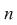this calculation may be tedious, or, indeed, impractical.

An easier method is to use de Moivre's formula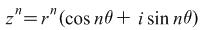.

## 4.2  Example

Calculate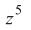where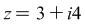.

Solution:

The modulus ofis found by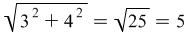.

The argument of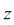is found by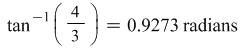(noting that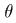is in the first quadrant).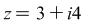can therefore be re-written as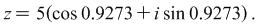Using de Moivre's formula,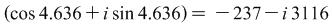.

# 5  Roots of complex numbers

Roots of complex numbers are also found using de Moivre's formula, this time in the form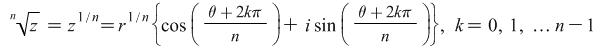.

When dealing with real numbers, we are used to there being a maximum of two solutions when calculating roots i.e. two solutions,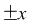, for even roots, and one solution,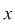, for odd roots. However, when calculating complex roots, there are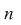different solutions forroots.

## 5.1  Examples

i) Find the complex 4th roots of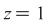.

ii) Find the complex 3rd (cube) roots of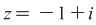.

i) Solution:

The modulus ofis clearly given by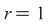. The argument of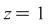is zero, sincelies on the positive real axis. The complex 4th roots ofare therefore given by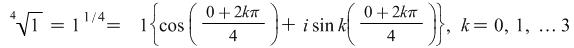i.e. the roots are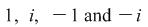. The results can easily be verified by calculation.

ii) Solution: The modulus of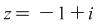is given by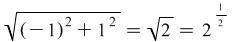.

The argument ofis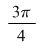, sincelies in the 2nd quadrant of the Argand diagram.

The complex cube roots ofare therefore given by